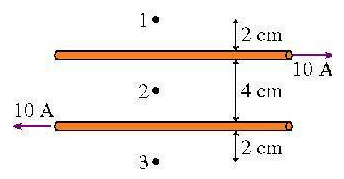# Problem: a) What is the magnetic field strength at point 1?b) What is the magnetic field strength at point 2?c) What is the magnetic field strength at point 3?

###### FREE Expert Solution

Magnetic field around a wire carrying current:

$\overline{){\mathbf{B}}{\mathbf{=}}\frac{{\mathbf{\mu }}_{\mathbf{0}}\mathbf{i}}{\mathbf{2}\mathbf{\pi }\mathbf{r}}}$

Right-hand rule:

Grab the conductor with the right hand:

The thumb points in the direction of the current, the fingers curl around the conductor in the direction of the magnetic field.

a)

The magnetic field strength at point 1 is the sum of the fields due to the two wires. The fields are directed in opposite directions. The field due to the top wire is out of the page while the field due to the lower wire is into the page.

We'll take out of the page as the positive direction. The top wire is 1 and the lower wire is 2:

$\begin{array}{rcl}{\mathbf{B}}_{\mathbf{net}}& \mathbf{=}& {\mathbf{B}}_{\mathbf{1}}\mathbf{-}{\mathbf{B}}_{\mathbf{2}}\\ & \mathbf{=}& \frac{{\mathbf{\mu }}_{\mathbf{0}}{\mathbf{i}}_{\mathbf{1}}}{\mathbf{2}{\mathbf{\pi r}}_{\mathbf{1}}}\mathbf{-}\frac{{\mathbf{\mu }}_{\mathbf{0}}{\mathbf{i}}_{\mathbf{2}}}{\mathbf{2}{\mathbf{\pi r}}_{\mathbf{2}}}\\ & \mathbf{=}& \frac{{\mathbf{\mu }}_{\mathbf{0}}}{\mathbf{2}\mathbf{\pi }}\mathbf{\left(}\frac{{\mathbf{i}}_{\mathbf{1}}}{{\mathbf{r}}_{\mathbf{1}}}\mathbf{-}\frac{{\mathbf{i}}_{\mathbf{2}}}{{\mathbf{r}}_{\mathbf{2}}}\mathbf{\right)}\end{array}$

μ0 = 4π × 10−7 H/m (permeability constant).

i1 = 10A

i2 = 10A###### Problem Detailsa) What is the magnetic field strength at point 1?

b) What is the magnetic field strength at point 2?

c) What is the magnetic field strength at point 3?